﻿ 低码率扩频通信LoRa传输距离分析及数据测试分析

# 低码率扩频通信LoRa传输距离分析及数据测试分析Analysis of LoRa Transmission Distance and Data Test in Low Bit Rate Spread Spectrum Communication

Abstract: In view of LoRa low bit rate spread spectrum communication, this paper first analyzes the spatial transmission of wireless communication, and analyzes the relevant factors of transmission distance in the transmission process. Firstly, the relationship between the distance d between the receiving and transmitting antennas and the transmitting power of the transmitter, the sensitivity of the receiver, the gain of the transmitting antenna, the gain of the receiving antenna, the carrier frequency, the insertion loss of the base station’s transmitting antenna’s feeders and the air transmission loss caused by the environment is analyzed in the ideal environment, so as to theoretically analyze the influencing factors of the transmission distance. The basic principle of Lora spread spectrum communication is analyzed and compared with FSK modulation. Finally, through a large number of tests, transmission distance, packet loss rate, signal quality, signal-to-noise ratio index are analyzed, and the comparison results between the actual measurement and the theory are obtained.

1. 引言

LoRa  是一种基于扩频技术的远距离无线传输技术，其实也是诸多LPWAN通信技术中的一种，主要面向物联网  (IoT)或者M2M等应用。为用户提供一种简单的能实现远距离、低功耗的无线通信手段。LoRa的优势在于长距离通信能力。单个网关或基站可以覆盖整个城市或数百平方公里范围。在一个给定的位置，距离在很大程度上取决于环境或障碍物，但LoRa有一个链路预算优于其他任何标准化的通信技术 。

LoRa是物理层或无线调制用于建立长距离通信的链路。许多传统的无线系统使用频移键控(FSK)调制作为物理层 ，它是一种实现低功耗的非常有效的调制。LoRa是基于线性调频扩频调制，它保持了像FSK调制相同的低功耗特性，但明显地增加了通信距离。但是很多时候用户在使用过程中，与实际标称的传输距离差别很大。如理论上采用低码率传输，可以传输15 kM，但是受一些因素的影响，在工程应用中仅仅是5 KM左右。因此本文首先对无线通信的空间传输进行分析，通过链路预算在给定的环境中决定距离的主要因素。分析LoRa扩频通信的基本原理，将其与FSK调制进行对比其性能特点。最终通过大量的试验测试，对传输距离、丢包率、信号质量、信噪比指标进行分析，得到实测与理论的对比结果。

2. LoRa通信的扩频通信原理

2.1. 通信带宽分析

$C=W\ast {\mathrm{log}}_{2}\left(1+\frac{S}{N}\right)$ (1)

S——有用信号功率；

W——频带宽度；

N——噪声功率。

${G}_{P}=\frac{W}{\Delta F}$ (2)

${M}_{J}={G}_{P}-\left[\left(\frac{S}{N}\right)+{L}_{S}\right]$ (3)

2.2. LoRa扩频通信传输速率分析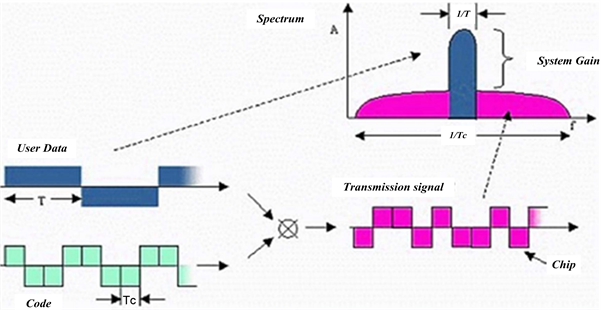Figure 1. Principle of LoRa spread spectrum communication

3. LoRa扩频通信传输距离分析

LoRa扩频调制的示意图如上所示，用户数据的原始信号与扩展编码位流进行XOR (异或)运算，生成发送信号流，这样，提高了接收灵敏度。

${P}_{t}-{P}_{r}+{G}_{t}+{G}_{r}=20\mathrm{log}\frac{4\pi fd}{c}+{L}_{c}+{L}_{0}$ (4)

Pr——接收器的灵敏度，单位dBm；

Gt——发射天线增益，单位dB；

Gr——接收天线增益，单位dB；

f——载波频率，单位Hz；

d——收、发天线间距离；

c——光速(3 × 108m/s)；

Lc——基站发射天线的馈线插损，单位dB；

L0——因环境带来的空中传播损耗，单位dB。

${P}_{t}-{P}_{r}+{G}_{t}+{G}_{r}=20\mathrm{log}f+20\mathrm{log}d+{L}_{c}+{L}_{0}-147.56\left(\text{dB}\right)$ (5)

$d={10}^{\frac{{P}_{t}-{P}_{r}+{G}_{t}+{G}_{r}-{L}_{c}-{L}_{0}-20\mathrm{log}f+147.56\text{\hspace{0.17em}}\text{dB}}{20}}$ (6)

4. LoRa长距离通信测试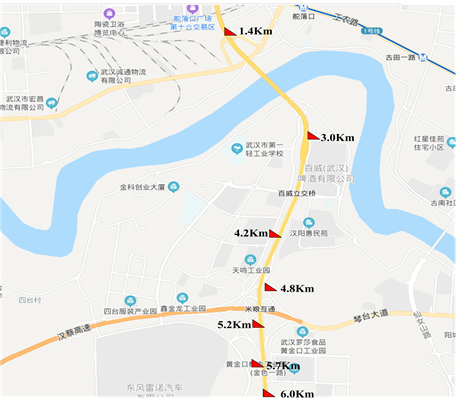Figure 2. Layout of multi point distance measurement between LoRa terminal and LoRawan gateway

$\text{SNR}=\frac{{P}_{\text{signal}}}{{P}_{\text{noise}}}={\left(\frac{{A}_{\text{signal}}}{{A}_{\text{noise}}}\right)}^{2}$ (7)

$\text{SNR}\left(\text{dB}\right)=10\mathrm{lg}\frac{{P}_{\text{signal}}}{{P}_{\text{noise}}}=20\mathrm{lg}{\left(\frac{{A}_{\text{signal}}}{{A}_{\text{noise}}}\right)}^{2}$ (8)

${P}_{\text{noise}}$ 为噪声功率(Power of Noise)

${A}_{\text{signal}}$ 为信号幅度(Amplitude of signal)

${A}_{\text{noise}}$ 为噪声幅度(Amplitude of Noise)

$x\left(\text{dBm}\right)=10\mathrm{lg}\frac{P\left(\text{mW}\right)}{1\left(\text{mW}\right)}$ (9)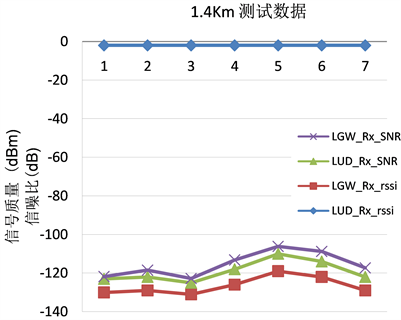Figure 3. SNR of LoRa test at 1.4 km point and signal strength rssi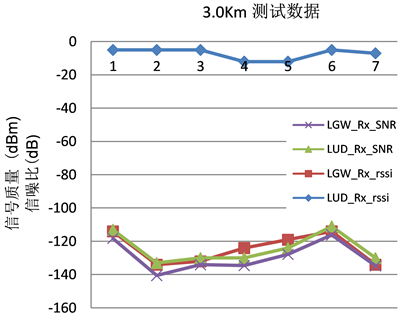Figure 4. SNR of LoRa test at 3.0 km point and signal strength rssi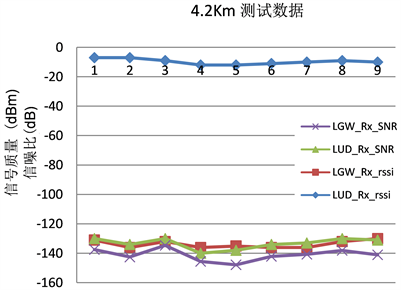Figure 5. SNR of LoRa test at 4.2 km point and signal strength rssi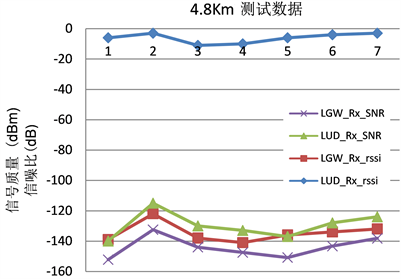Figure 6. SNR of LoRa test at 4.8 km point and signal strength rssi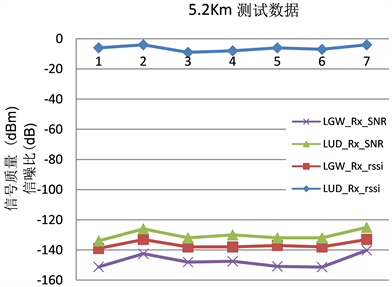Figure 7. SNR of LoRa test at 5.2 km point and signal strength rssiFigure 8. SNR of LoRa test at 5.7 km point and signal strength rssi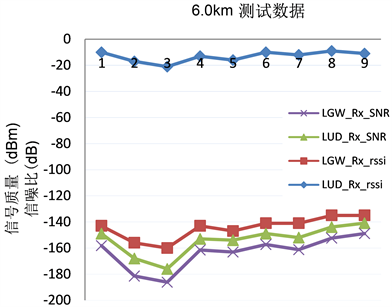Figure 9. SNR of LoRa test at 6.0 km point and signal strength rssiTable 1. SNR measurement averageTable 2. Signal strength measurement average RSSITable 3. Packet loss rate measurement LOS

5. 总结

 Zhang, X.L., Luan, Y.G. and Zhang, W.J. (2017) Research on the Application of LoRa Wireless Technology in Digital Construction of Oil Fields. Oil & Gas Field Surface Engineering, 36, 60-62, 66.

 Zhang, N.L., Zhang, R., Chen, L., et al. (2019) Status Monitoring System for Heavy Oil Injection Production Plunger Pump Based on Internet of Things. Journal of Xi’an Shiyou University (Natural Science Edition), 34, 112-116.

 Li, M.Z., Zi, W.B. and Wang, H. (2019) Research on MAC Layer TDMA Time Slot Allocation Protocol in LoRa Wireless Network. Computer Engineering, 45, 95-99, 118.

 Bor, M.C., Roedig, U. and Voigt, T. (2016) Do LoRa Low-Power Wide-Area Networks Scale? Pro-ceeding of the 19th ACM International Conference of Modeling, Analysis and Simulation of Wireless and Mobile Sys-tem-MSWiM’16 Malta, Malta, 59-67.
https://doi.org/10.1145/2988287.2989163

 Rizzi, M., Ferrari, P., Flam-mini, A., Sisinni, E. and Gidlund, M. (2017) Using LoRa for Industrial Wireless Networks. 2017 IEEE 13th internation-al Workshop on Factory Communication System (WFCS), Trondheim, Norway, 31 May-2 June 2017, 1-4.
https://doi.org/10.1109/WFCS.2017.7991972

 Cheong, P.S., Bergs, J., Hawinkel, C., et al. (2017) Comparison of LoRa WAN Classes and Their Power Consumption. IEEE Symposium on Communications and Vehicular Technology (SCVT), Leuven, 14-14 November 2017, 1-6.
https://doi.org/10.1109/SCVT.2017.8240313

 Rai, A., Chintalapudi, K.K., Padmanabhan, V.N., et al. (2012) Zee: Zero-Effort Crowdsourcing for Indoor Localization. Proceedings of the 18th Annual International Conference on Mobile Computing and Networking (MOBICOM), Istanbul, 293-304.
https://doi.org/10.1145/2348543.2348580

 Goyal, P., Ribeiro, V.J., Saran, H., et al. (2011) Strap-Down Pe-destrian Dead-Reckoning System. IEEE International Conference on Indoor Positioning & Indoor Navigation, Guimaraes, 21-23 September 2011, 1-7.
https://doi.org/10.1109/IPIN.2011.6071935

Top